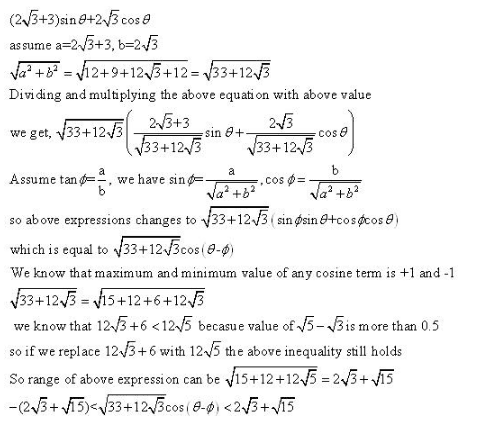RD Sharma Solutions Class 11 Trigonometric Ratios Of Compound Angles Exercise 7.2

RD Sharma Solutions Class 11 Chapter 7 Exercise 7.2

Trigonometric Ratios of Compound Angles Ex 7.2 Q1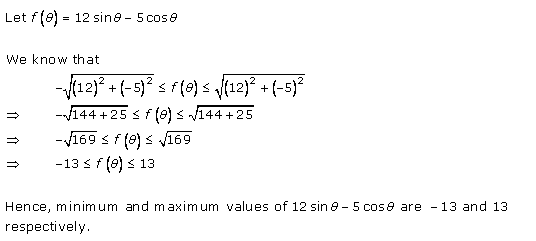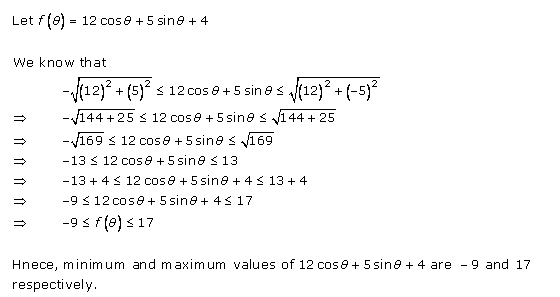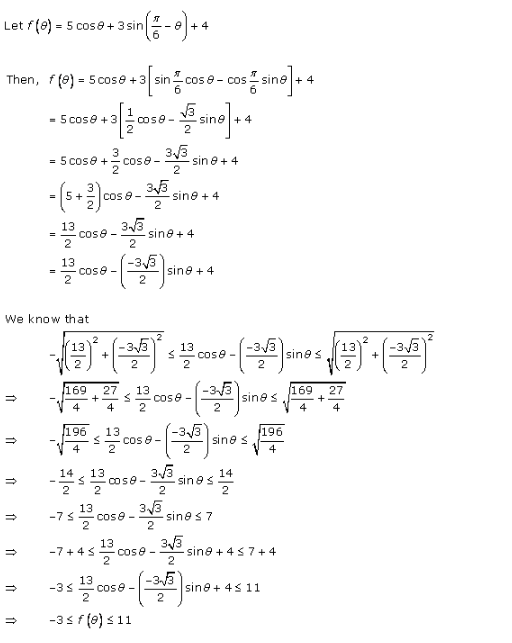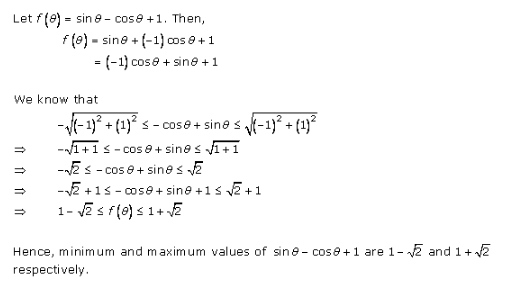Trigonometric Ratios of Compound Angles Ex 7.2 Q2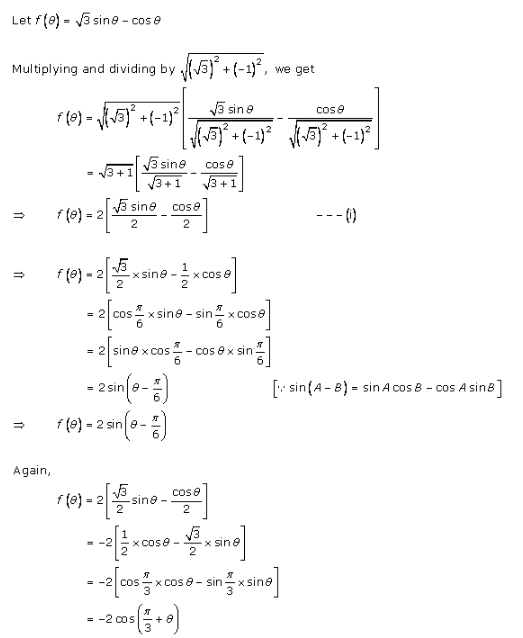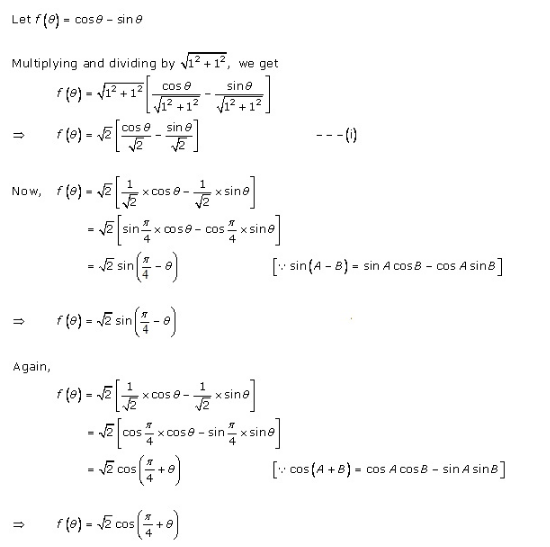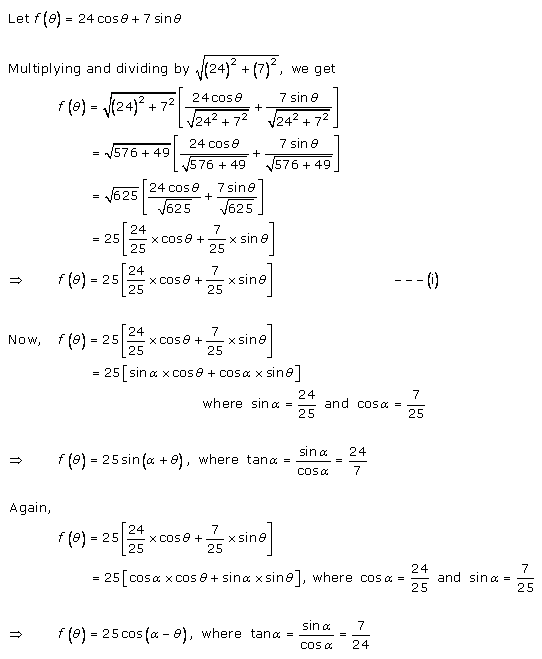Trigonometric Ratios of Compound Angles Ex 7.2 Q3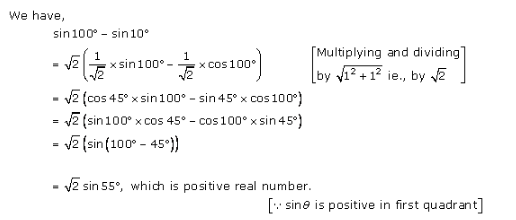Trigonometric Ratios of Compound Angles Ex 7.2 Q4